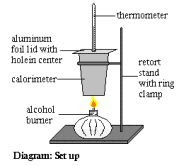# Determining Heat of Combustion Using Calorimetry

 Date 12.05.2017 Size 16.66 Kb. #19548

## Determining Heat of Combustion Using Calorimetry

Materials:
The following is the list of materials and equipment used in this experiment:

• Alcohol burner (containing ethanol)

• Approximately 100 mL of water

• Retort stand (with ring clamp & collar)

• Calorimeter with lid (aluminum foil was used for the lid)

• Thermometer

• 100 mL graduated cylinder

• Balance

Procedure:

• First the alcohol burner was massed, followed by the calorimeter (as well as its lid).

• Next about 100 ml of water was added to the calorimeter and the calorimeter and the water were massed together (to determine the mass of the water).

• The apparatus was set up as shown in the diagram on the left.

• The temperature of the water was taken to the nearest half a degree.

• The alcohol burner was then ignited and the temperature of the water was allowed to rise about 40°C before the burner was put out. The highest temperature obtained by the water was recorded.

• The alcohol burner was massed again to determine how much alcohol had been consumed.

• Assuming that the heat lost by the ethanol fuel equaled the heat gained by the water and the calorimeter, the heat of combustion of ethanol was determined.Observations:
The ethanol burnt with an orange-yellow flame, showing that incomplete combustion was taking
place. The temperature rose rapidly, increasing from an initial temperature of 22°C to a maximum of 65°C. The mass of the alcohol burner decreased during the combustion as alcohol was burnt up. The alcohol burner was originally 275.61 g. After burning it was massed as 273.63 g.

Calculations:
The following table contains the measurements taken during this experiment.
 Type of Measurement Measurement Mass of alcohol burner before combustion 275.61 g Mass of alcohol burner after combustion 273.63 g Mass of calorimeter (without lid) 54.12 g Mass of calorimeter (without lid) and water 152.59 g Mass of Aluminum foil lid 2.13 g Temperature of water before combustion 22.0 °C Temperature of water after combustion 65.0 °C

Mass of water = mass of calorimeter and water – mass of calorimeter

= 152.59 – 54.12

= 98.47
Heat gained by water = m ?T C

= (98.47 g) (65.0°C – 22.0°C) (4.18 J/g°C)

= 17.698 kJ

Heat gained by calorimeter = m ?T C

= (54.12 + 2.13) (65.0°C – 22.0°C) (0.9 J/g°C)

= 2.177 kJ
Total Heat given off by ethanol = 17.698 kJ + 2.177 kJ

= 19.875
Energy given off per gram of ethanol = Total Heat given off / mass used up

= 19.875 kJ / (275.61g – 273.63g)

= 10.038 kJ/g

= 10.0 kJ/g
Energy given off per mole of ethanol = Energy given off per gram X molar mass

= 10.038 kJ/g X 46.08 g/mol

= 463 kJ/mol
The purpose of this lab was to determine the energy given off per gram and per mole
of ethanol fuel. It was found that ethanol gives off 10.0 kJ/g and 463 kJ/mol.